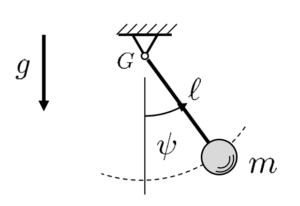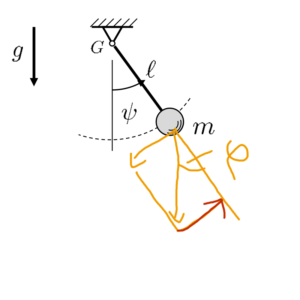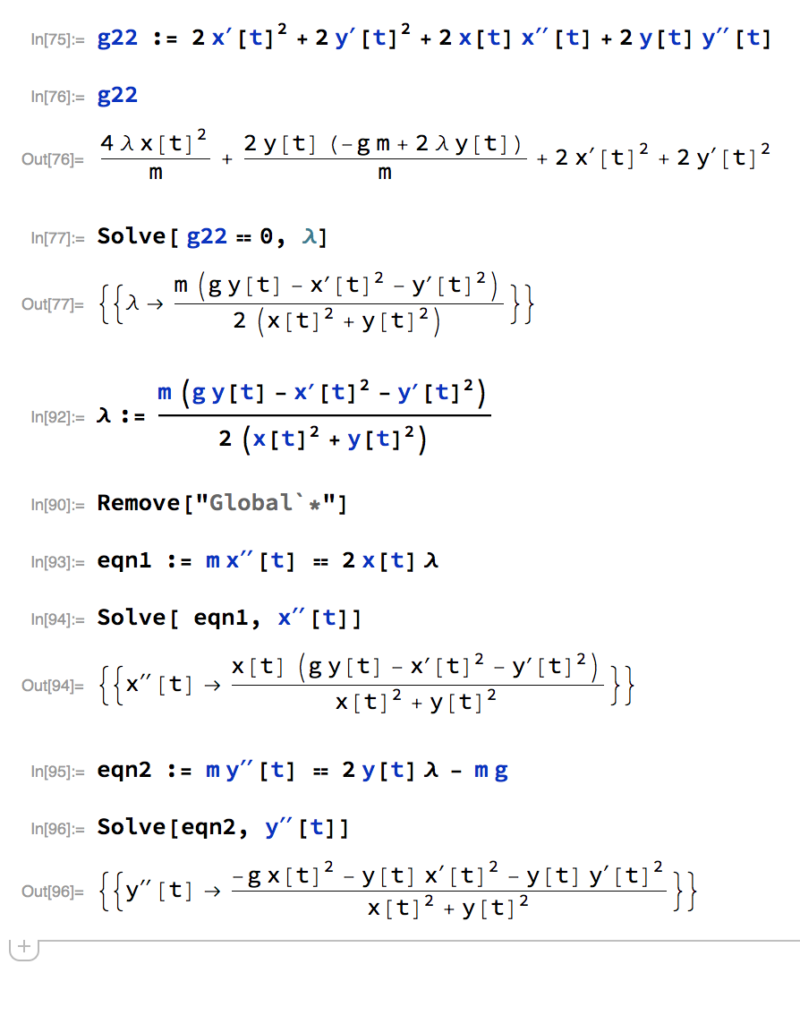Math Monkey

φI study mechanical engineering, as anyone who knows me know. I’m pretty bad at it. Hence this website….One thing I’ve noticed, and discussed with others is the entire lack of…speciality in subjects, or specifically. explanations of things. Any Google-fu’er can tell you, the more indepth you get into a subject the harder it can be to find real answers. A Recent subject for me, while looking into/researching vibration engineering for a 5 axis laser cutter I’ve been working on was “reactionary forces“. Often described by Newtons impulsesatz as $$m\: \ddot{x} = \displaystyle\sum_{i=1}^{n}\,F_i + Z_i$$ Where $Z_i$ is the reactionary force. I’m the last person in the world…or well maybe second last who should be asked what its all about. But basically to conserve energy in a system, you need it…but it doesn’t really exist. ‘Cause it’s virtual or some bullshit.

Anyways, it’s something that I’ve only ever had to calculate once, for a single assignment, and never had to do again. So I figured I’d write a little about it. Mostly because it was frustrating, but interesting at the same time. This is math heavy post btw.

The Mathematical PendulumThis is a system that most people are pretty familiar with. In physics class everywhere its used to show all sorts of different things, Like how to calculate the speed of the mass in $\mathrm{T_E} = \frac{1}{2}mv^{2}-mgl(1-\cos{\phi})$ or things like how the period…time it takes for the pendel to swing has nothing to do with its mass, and only its length in $$\mathrm{T} = 2\pi\sqrt{\frac{l}{g}}$$. The only problem with these methods…is that the pendulum doesn’t actually swing harmonically. Which means the Force exerted on the system isn’t proportional to the angle. Bummer

Once you start getting into higher mechanics the pendulum starts getting more complicated…and of course more accurate in determining its movements. One of the ways describing it’s movement, is using the

SSJ2 Lagrange

Which is basically a lot of magic that in short order describes the movement of the pendulum, as shown;

First we describe the system using Energie erhaltungssatz and potentialle energie;

$$\mathrm{E}=\frac{1}{2}m(l\dot{\phi})^{2}\:\:\mathrm{U}=\mathrm{mgl}\cos{\phi}$$ After this we stick it in the the Lagrange function being, $$\mathrm{L} = E-U$$ $$\mathrm{L} = \frac{1}{2}m(l\dot{\phi})^{2}-\mathrm{mgl}\cos{\phi}$$ Now we Comes the mathemagics. We have to do some partial derivatives on φ.

$$\frac{d}{dt}\frac{\delta\mathrm{L}}{\delta\mathrm{\dot{\phi}}} = ml^{2}\ddot{\phi} + \mathrm{0}$$ and

$$\frac{\delta\mathrm{L}}{\delta\mathrm{\phi}}= -mgl\sin{\phi}$$ Now we get into more randomness…namely the virtual force. Q!

$$\mathrm{Q}=\frac{d}{dt}\frac{\delta\mathrm{L}}{\delta\mathrm{\dot{\phi}}}-\frac{\delta\mathrm{L}}{\delta\mathrm{\phi}} =ml^{2}\ddot{\phi}+mgl\sin{\phi}$$

we can now say, with Q = 0$$\Rightarrow \ddot{\phi} + \frac{g}{l}\sin{\phi} = 0$$ is the movement function of our system…and after linearisation we can describe with relatively high accuracies (around 30 degrees apparently +/-) our system.

But wait! Theres more!

We can use the Drallsatz.

$$(\Theta_{eig}+\Theta_{str})\dot{\omega}=\mathrm{M_{Gz}}\:\Rightarrow \ddot{\phi}(0+(x^{2}+y^{2})\mathrm{m}=-mgl\sin{\phi}\:\Rightarrow\ddot{\phi} + \frac{g}{l}\sin{\phi} = 0$$ Huh, that was quick. Buuut not quite so easy to see…especially the sin(φ) part of it..

The $\mathrm{M_{Gz}}$ is actually describing the moment around the Lager point G…Moment as a force  ⊥ mass × length..But our only working force is gravity…However if we have a little look, we can see gravity can be described as a function of φ, like soThere is also the Energy Conservation Act Function

$$\dot{E}+\dot{U}= 0\:\Rightarrow\:\frac{d}{dt}(\frac{1}{2}ml^{2}\dot{\phi^{2}})+\frac{d}{dt}(-mgl\cos{\phi}) = 0\:\Rightarrow\:ml^{2}\dot{\phi}(\ddot{\phi}+\frac{g}{l}\sin{\phi} = 0$$

As you can see…there are atleast two methods that make finding the equation of a pendulum pretty quick and easy….Why choose Lagrange?

Well if you remember at the beginning of the post, we actually we looking for the Zwangskraft. Which unfortunately, none of these methods describe…except for Lagrange. So, How does that work?

SSJ Lagrange

So we need to describe our system nach Newton, with our Ansatz being;

$$m\ddot{r}=\mathrm{F_g}+\mathrm{Z}$$

and the Zwangskraft Ansatz

$$\mathrm{Z_i}=\lambda_i(t)\,\nabla g_i(r,t)$$

So our Zwangskraft is a reduced Freiheitsgrad system, Infact the entire idea is to reduce the free moving points so it makes the system easier to describe. We have $S(3)-F(2)=1$ 3 movement possibilities, and we’ll have two constraints on the system…which gives us a single Freiheitsgrad Which is awesomely easy to solve.

So just to keep in mind, the Zwangs kraft $\mathrm{Z_i}$ is orthogonal to our system shown in $\mathrm{Z_i}\bot\nabla\mathrm{g_i}(r,t)$ and $\lambda_i$ is our Lagrange operator. Those two mulitplied with each other gives us our Zwangskraft.

So we build Lagrange first form with $$m\ddot{r}= \mathrm{F}+\displaystyle\sum_{i}^{n}\lambda_i(t)\,\nabla g_i(r,t) \Rightarrow (3+n)\text{equations, x, y, z, and }\lambda_1\text{,}\lambda_2$$

Our receipe for calculating such forces can be listed as so;

• Build Constraints  $g_i(r,t)=0$
• Calculate the gradient of said constraints $\nabla\,g_i(r,t)$
• Build the Lagrange function
• Solve the DGL.

So back to the Pendulum.

Step one

We will use$$\text{4)}\,g_1 :=z(t) = 0$$ $$\text{5)}\,g_2 :=x^{2}+y^{2}-l^{2}=0$$

Step two

$$\nabla\,g_1 = [0,0,1]^{\mathrm{T}}$$  $$\nabla\,g_1 = [2x,2y,0]^{\mathrm{T}}$$

Step three

$$\text{1)}\,m\ddot{x}=\lambda_2\,2x$$$$\text{2)}\,m\ddot{y}=\lambda_2\,2y-mg$$$$\text{3)}\,m\ddot{z}=\lambda_1$$

Step four

Now it’s time to start stickin’ shit inside of each other and solve. If we rearrange our equations 1, 2, and 3. and put in our 4, and 5 in our formulas, we can solve for our lambda. We’ll have to first do two derivatives of 5 $$\frac{dg_2}{dt}= 2x(t)\dot{x}(t)+2x(t)\dot{y}(t)$$ and $$\frac{dg_2}{dtt}2\dot{x}^{2}+2x(t)\ddot{x}+2\dot{y}^{2}+2y(t)\ddot{y}(t)$$   Now we can put our new 1 and 2 in 5. Once we do that, we get and solve for λ we get

$$\lambda_2 := \frac{m(g\,y(t)-\dot{x}(t)^{2}-\dot{y}(t)^{2})}{2(x(t)^{2}+y(t)^{2})}$$ we can see quickly that $\lambda_1$ is equal to zero if one follows the same route as for $g_2$ and sets $\lambda_1$ in the second derivative of $g_1$.

Now for the last final thing, we put lambda back into 1 and 2 and we get our Zwangskraft

$$\ddot{x}(t) = \frac{x(t)(g\,y(t)-\dot{x}(t)^{2}-\dot{y}(t)^{2})}{L^{2}}$$

$$\ddot{y}(t) = \frac{x(t)(-g\,x(t)^{2}-y(t)(\dot{x}(t)^{2}-\dot{y}(t)^{2})}{L^{2}}$$

Phew that was a long one. But I remembered a lot..and it gave me an excuse to play with mathematica. Here’s the results;